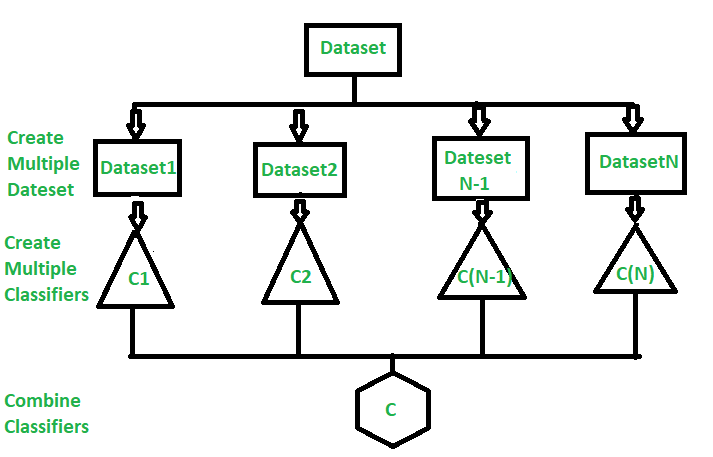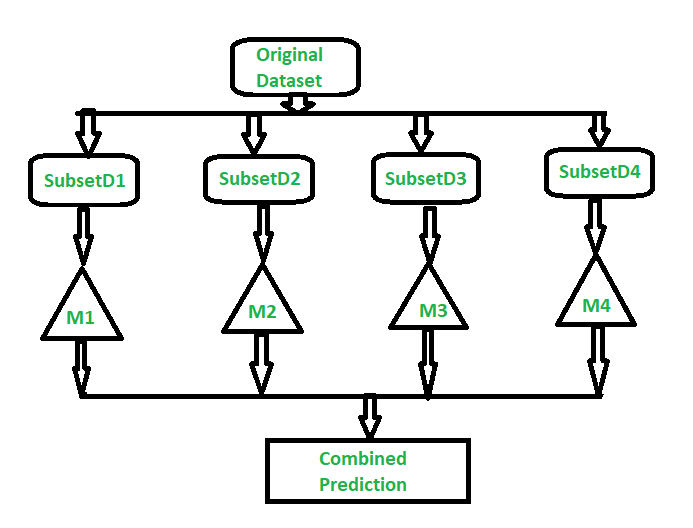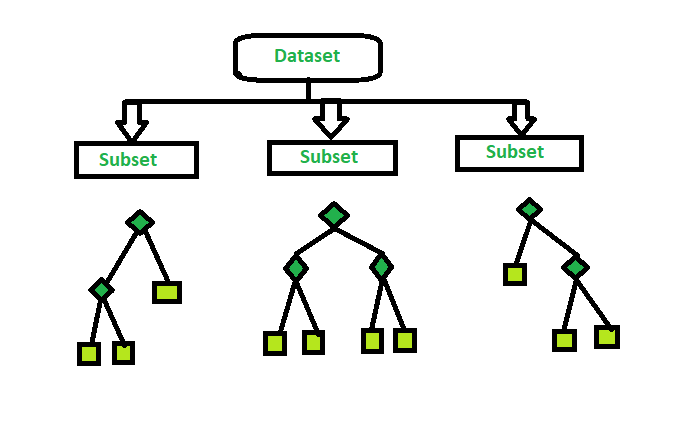Related Articles
Ensemble Classifier | Data Mining
• Difficulty Level : Easy
• Last Updated : 30 May, 2019

Ensemble learning helps improve machine learning results by combining several models. This approach allows the production of better predictive performance compared to a single model. Basic idea is to learn a set of classifiers (experts) and to allow them to vote.

Advantage : Improvement in predictive accuracy.
Disadvantage : It is difficult to understand an ensemble of classifiers.Why do ensembles work?

Dietterich(2002) showed that ensembles overcome three problems –

• Statistical Problem –
The Statistical Problem arises when the hypothesis space is too large for the amount of available data. Hence, there are many hypotheses with the same accuracy on the data and the learning algorithm chooses only one of them! There is a risk that the accuracy of the chosen hypothesis is low on unseen data!
• Statistical Problem –
The Computational Problem arises when the learning algorithm cannot guarantees finding the best hypothesis.
• Representational Problem –
The Representational Problem arises when the hypothesis space does not contain any good approximation of the target class(es).

Main Challenge for Developing Ensemble Models?

The main challenge is not to obtain highly accurate base models, but rather to obtain base models which make different kinds of errors. For example, if ensembles are used for classification, high accuracies can be accomplished if different base models misclassify different training examples, even if the base classifier accuracy is low.

Methods for Independently Constructing Ensembles –

• Majority Vote
• Bagging and Random Forest
• Randomness Injection
• Feature-Selection Ensembles
• Error-Correcting Output Coding

Methods for Coordinated Construction of Ensembles –

• Boosting
• Stacking

Reliable Classification: Meta-Classifier Approach
Co-Training and Self-Training

Types of Ensemble Classifier –

Bagging:
Bagging (Bootstrap Aggregation) is used to reduce the variance of a decision tree. Suppose a set D of d tuples, at each iteration i, a training set Di of d tuples is sampled with replacement from D (i.e., bootstrap). Then a classifier model Mi is learned for each training set D < i. Each classifier Mi returns its class prediction. The bagged classifier M* counts the votes and assigns the class with the most votes to X (unknown sample).

Implementation steps of Bagging –

1. Multiple subsets are created from the original data set with equal tuples, selecting observations with replacement.
2. A base model is created on each of these subsets.
3. Each model is learned in parallel from each training set and independent of each other.
4. The final predictions are determined by combining the predictions from all the models.
5.Random Forest:
Random Forest is an extension over bagging. Each classifier in the ensemble is a decision tree classifier and is generated using a random selection of attributes at each node to determine the split. During classification, each tree votes and the most popular class is returned.

Implementation steps of Random Forest –

1. Multiple subsets are created from the original data set, selecting observations with replacement.
2. A subset of features is selected randomly and whichever feature gives the best split is used to split the node iteratively.
3. The tree is grown to the largest.
4. Repeat the above steps and prediction is given based on the aggregation of predictions from n number of trees.
5.